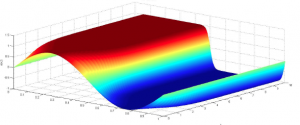# Parallel Spectral Numerical MethodsTerm:
2012
Published:
April 24, 2012
Revised:
June 5, 2015

Parallel Spectral Numerical Methods starts by taking a quick look at finite precision arithmetic. It then discusses how to solve ordinary differential equations (ODE) and partial differential equations (PDE) using separation of variables. Next, it introduces numerical time-stepping schemes that can be used to solve ODEs and PDEs. This is followed by an introduction to pseudo spectral methods through an overview of the discrete Fourier Transform (DFT) and the Fast Fourier Transform (FFT) algorithm that is used to quickly calculate the DFT. Finally it will combine all of these to solve a couple of different PDEs first in a serial setting and then in a parallel setting.

The programs will use Matlab and Fortran. A Python implementation of some of the Matlab programs is also provided.

dScribe: Surong Ruan

This resource is supported by a level I LSA Instructional Technology Committee Grant

Gong Chen is an undergraduate student in School of Mathematics, University of Minnesota- Twin Cities. His mathematical interests are analysis, differential equations and mathematical physics.

Brandon Cloutier is a physics student at the University of Michigan. He is interested in convection and the impact of storms on mixing and tracers in the ocean.

Ning Li works for the Numerical Algorithms Group as a Technical Consultant. He specializes in numerical software development, in particular in the area of high performance computing. He authors the parallel software framework 2DECOMP&FFT.

Benson Muite was a postdoctoral assistant professor at the University of Michigan. His research interests are partial differential equations and high performance computing.

Paul Rigge is an electrical engineering and computer science undergraduate. He is interested in signal processing and communications.

Sudarshan Balakrishnan is an honors mathematics, physics and statistics student at the University of Michigan. He is interested in number theory.

Andre Souza is a mathematics graduate student at the University of
Michigan. He is interested in convection.

Jeremy West is a PhD candidate in the Department of Mathematics. His research focuses on discrete mathematics, information theory, and theoretical computer science.Term:
2012
Published:
April 24, 2012
Revised:
June 5, 2015

### Texts

Parallel Spectral Numerical Methods: Code and Source Files on GitHub

Gong Chen
Brandon Cloutier
Ning Li
Benson Muite
Paul Rigge

Parallel Spectral Numerical Methods: Full Text

Gong Chen
Brandon Cloutier
Ning Li
Benson Muite
Paul Rigge

Parallel Spectral Numerical Methods: Wikibooks Version

Gong Chen
Brandon Cloutier
Ning Li
Benson Muite
Paul Rigge